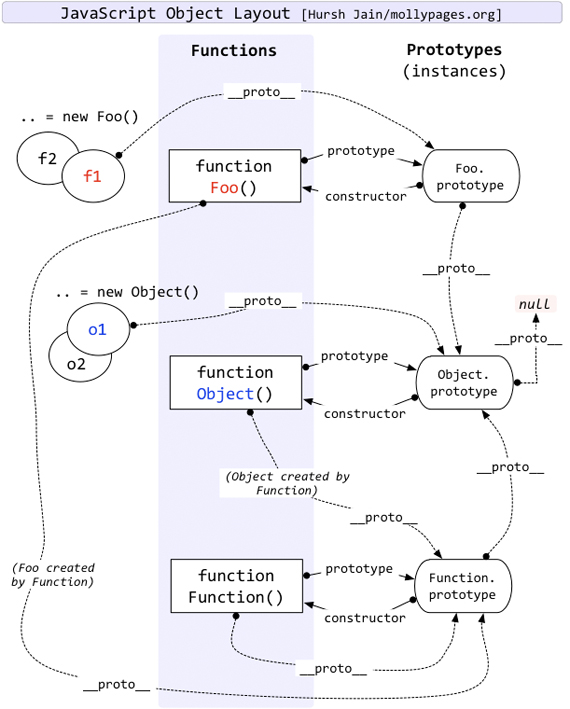# JavaScript instanceof 运算符深入剖析

IBMdW 发布于 2013/06/14 14:31

instanceof 运算符简介

 ```var oStringObject = new String("hello world"); console.log(oStringObject instanceof String); // 输出 "true"```

instanceof 运算符的常规用法

 ```// 判断 foo 是否是 Foo 类的实例 function Foo(){} var foo = new Foo(); console.log(foo instanceof Foo)//true```

 ```// 判断 foo 是否是 Foo 类的实例 , 并且是否是其父类型的实例 function Aoo(){} function Foo(){} Foo.prototype = new Aoo();//JavaScript 原型继承 var foo = new Foo(); console.log(foo instanceof Foo)//true console.log(foo instanceof Aoo)//true```

 ```console.log(Object instanceof Object);//true console.log(Function instanceof Function);//true console.log(Number instanceof Number);//false console.log(String instanceof String);//false console.log(Function instanceof Object);//true console.log(Foo instanceof Function);//true console.log(Foo instanceof Foo);//false```

 ```11.8.6 The instanceof operator The production RelationalExpression: RelationalExpression instanceof ShiftExpression is evaluated as follows: 1. Evaluate RelationalExpression. 2. Call GetValue(Result(1)).// 调用 GetValue 方法得到 Result(1) 的值，设为 Result(2) 3. Evaluate ShiftExpression. 4. Call GetValue(Result(3)).// 同理，这里设为 Result(4) 5. If Result(4) is not an object, throw a TypeError exception.// 如果 Result(4) 不是 object， //抛出异常 /* 如果 Result(4) 没有 [[HasInstance]] 方法，抛出异常。规范中的所有 [[...]] 方法或者属性都是内部的， 在 JavaScript 中不能直接使用。并且规范中说明，只有 Function 对象实现了 [[HasInstance]] 方法。 所以这里可以简单的理解为：如果 Result(4) 不是 Function 对象，抛出异常 */ 6. If Result(4) does not have a [[HasInstance]] method, throw a TypeError exception. // 相当于这样调用：Result(4).[[HasInstance]](Result(2)) 7. Call the [[HasInstance]] method of Result(4) with parameter Result(2). 8. Return Result(7). // 相关的 HasInstance 方法定义 15.3.5.3 [[HasInstance]] (V) Assume F is a Function object.// 这里 F 就是上面的 Result(4)，V 是 Result(2) When the [[HasInstance]] method of F is called with value V, the following steps are taken: 1. If V is not an object, return false.// 如果 V 不是 object，直接返回 false 2. Call the [[Get]] method of F with property name "prototype".// 用 [[Get]] 方法取 // F 的 prototype 属性 3. Let O be Result(2).//O = F.[[Get]]("prototype") 4. If O is not an object, throw a TypeError exception. 5. Let V be the value of the [[Prototype]] property of V.//V = V.[[Prototype]] 6. If V is null, return false. // 这里是关键，如果 O 和 V 引用的是同一个对象，则返回 true；否则，到 Step 8 返回 Step 5 继续循环 7. If O and V refer to the same object or if they refer to objects joined to each other (section 13.1.2), return true. 8. Go to step 5.```

 ```function instance_of(L, R) {//L 表示左表达式，R 表示右表达式 var O = R.prototype;// 取 R 的显示原型 L = L.__proto__;// 取 L 的隐式原型 while (true) { if (L === null) return false; if (O === L)// 这里重点：当 O 严格等于 L 时，返回 true return true; L = L.__proto__; } }```

JavaScript 原型继承机制```// 为了方便表述，首先区分左侧表达式和右侧表达式 ObjectL = Object, ObjectR = Object; // 下面根据规范逐步推演 O = ObjectR.prototype = Object.prototype L = ObjectL.__proto__ = Function.prototype // 第一次判断 O != L // 循环查找 L 是否还有 __proto__ L = Function.prototype.__proto__ = Object.prototype // 第二次判断 O == L // 返回 true```

 ```// 为了方便表述，首先区分左侧表达式和右侧表达式 FunctionL = Function, FunctionR = Function; // 下面根据规范逐步推演 O = FunctionR.prototype = Function.prototype L = FunctionL.__proto__ = Function.prototype // 第一次判断 O == L // 返回 true```

 ```// 为了方便表述，首先区分左侧表达式和右侧表达式 FooL = Foo, FooR = Foo; // 下面根据规范逐步推演 O = FooR.prototype = Foo.prototype L = FooL.__proto__ = Function.prototype // 第一次判断 O != L // 循环再次查找 L 是否还有 __proto__ L = Function.prototype.__proto__ = Object.prototype // 第二次判断 O != L // 再次循环查找 L 是否还有 __proto__ L = Object.prototype.__proto__ = null // 第三次判断 L == null // 返回 false```

 ```dojo.declare("Aoo",null,{}); dojo.declare("Boo",null,{}); dojo.declare("Foo",[Aoo,Boo],{}); var foo = new Foo(); console.log(foo instanceof Aoo);//true console.log(foo instanceof Boo);//false console.log(foo.isInstanceOf(Aoo));//true console.log(foo.isInstanceOf(Boo));//true```